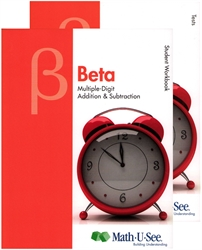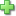# Math-U-See Beta - Student Pack

Publisher: Math-U-See
2012 Edition, ©2012, Publisher Catalog #1021
Hardcover
Price: \$48.00

See series description for full review.

Prerequisite: Complete the Alpha curriculum or master add and subtract facts.

• Lesson 01: Place Value and the Manipulatives
• Lesson 02: Sequencing; Word Problem Tips
• Lesson 03: Inequalities
• Lesson 04: Rounding to 10 and Estimation
• Lesson 05: Multiple-Digit Addition and Place Value Notation
• Lesson 06: Skip Count by 2
• Lesson 07: Addition with Regrouping (Carrying)
• Lesson 08: Skip Count by 10; 1 Penny = 1¢, 1 Dime = 10¢
• Lesson 09: Skip Count by 5; 5¢ = 1 Nickel
• Lesson 10: Money: Decimal Point and Dollars
• Lesson 11: Rounding to Hundreds; Multiple-Digit Addition with Regrouping
• Lesson 12: Adding Money; Mental Math
• Lesson 14: Measurement: 12 Inches = 1 Foot
• Lesson 15: Perimeter
• Lesson 16: Thousands and Place-Value Notation
• Lesson 17: Rounding to Thousands; Estimation
• Lesson 18: Multiple-Digit Column Addition; Mental Math
• Lesson 19: More Multiple-Digit Column Addition
• Lesson 20: Multiple-Digit Subtraction
• Lesson 21: Telling Time: Minutes; Mental Math
• Lesson 22: Subtraction with Regrouping (Borrowing)
• Lesson 23: Telling Time: Hours
• Lesson 24: Subtraction: Three-Digit Numbers; Mental Math
• Lesson 25: Ordinal Numbers; Tally Marks; Days and Months
• Lesson 26: Subtraction: Four-Digit Numbers
• Lesson 27: Subtraction: Money; Mental Math
• Lesson 28: Subtraction: Multiple-Digit Numbers
• Lesson 29: Reading Gauges and Thermometers
• Lesson 30: Bar Graphs and Line Graphs
• Appendix A: Identify Shapes; Fractional Parts
• Appendix B: Number Line

## Sample Lesson

Did you find this review helpful?
Series DescriptionClick here to write a review